﻿ 基于经验模态分解改进神经网络光伏出力预测
«上一篇文章快速检索 高级检索

 应用科技2020, Vol. 47Issue (3): 41-45  DOI: 10.11991/yykj.2019110180

### 引用本文QI Qi, CHEN Fangfang, XU Tianqi, et al. Improved neural network for output prediction of photovoltaic generation based on empirical modal decomposition[J]. Applied Science and Technology, 2020, 47(3): 41-45. DOI: 10.11991/yykj.201911018.### 文章历史

Improved neural network for output prediction of photovoltaic generation based on empirical modal decomposition
QI Qi, CHEN Fangfang, XU Tianqi, SUN Xiangsheng
School of Electrical and Information Technology, Yunnan Minzu University, Kunming 650504, China
Abstract: Photovoltaics power generation is a hot spot of research nowadays, and in the mean time, there are also shortcomings, such as instability and volatility of photovoltaic output. In this paper, a short-term power generation forecast model of genetic algorithm(GA)-BP neural network based on empirical modal decomposition(EMD) is proposed, which optimizes the defects of the BP neural network, such as too many times of iteration, long convergence time, etc. An EMD-GA-BP prediction model was established by obtaining power generation data from a small photovoltaic power station, and then compared with the single BP neural network prediction model and the GA-BP neural network prediction model, confirming that both the stability and error of the prediction model are small. This study has a certain research value.
Keywords: clean energy    PV generation    output forecasting    empirical modal decomposition    GA genetic optimization algorithm    BP neural network    combined forecast model

1 光伏发电输出功率影响因素

 ${x_i},{y_i} \in R$

 $r = \frac{{\displaystyle\sum\limits_{i = 1}^n {({x_i} - \overline x } )({y_i} - \overline y )}}{{{{\Bigg[\displaystyle\sum\limits_{i = 1}^n {({x_i}} - \overline x )^2}}\displaystyle\sum\limits_{i = 1}^n {({y_i}} - \overline y {)^2}\Bigg]^{1/2}}}$

2 建模原理 2.1 经验模态分解

1)假设原始信号为 ${x_{(t)}}$ ,找出局部极大值与极小值点，利用三次样条插值的办法，使上包络线与极大值连接，下包络值与极小值连接。

2)求上包络线与下包络线的均值 ${m_1}(t)$ ${x_1}(t)$ ${m_1}(t)$ 的差为

 ${h_1}(t) = {x_1}(t) - {m_1}(t)$

${h_1}(t)$ 为IMF，那么 ${h_1}(t)$ 为第一个分量。

3)如 ${h_1}(t)$ 不是IMF，则把它作为原始信号，重复步骤2)，得出

 ${h_{11}}(t) = {h_1}(t) - {m_{11}}(t)$

 ${h_{1k}}(t) = {h_{1(k - 1)}}(t) - {m_{1k}}(t)$

4)从 ${x_{(t)}}$ 中分离出 ${c_1}(t)$ ${c_1}(t)$ 是原始信号的第一个IMF成分, 代表 $x(t)$ 最高频率的分量，得到：

 ${r_1}(t) = x(t) - {c_1}(t)$

${r_1}(t)$ 为新的信号，然后把 ${r_1}(t)$ 当作原始信号重复步骤1)~步骤3)，得到第2个IMF的 ${c_2}(t)$ ，代表 ${r_1}(t)$ 的最高频率分量，重复 $n$ 次得到：

 ${r_2}(t) = {r_1}(t) - {c_2}(t)$
 ${r_n}(t) = {r_{n - 1}}(t) - {c_n}(t)$

${r_n}(t)$ 为一个极小值常量时，停止分解，得到：

 $x(t) = \sum\nolimits_{i = 1}^n {{c_i}} (t) + {r_n}(t)$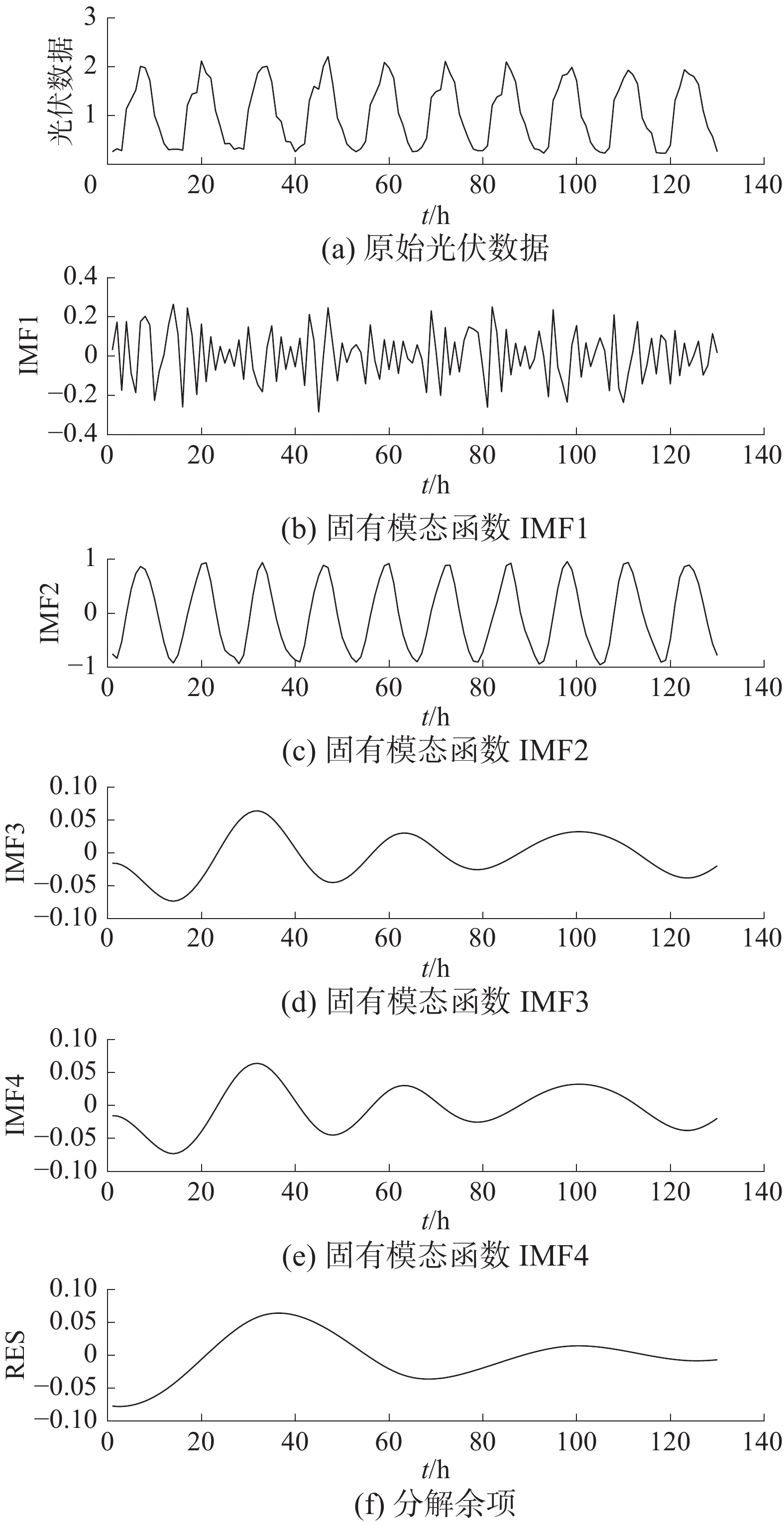Download: 图 1 EMD分解

2.2 BP神经网络模型

BP神经网络的整个学习过程为信号正向传播和误差的方向回传。假设训练样本数为 $N$ ，最大训练次数为 $T$ , $\omega (t)$ 为第 $t$ 次迭代的权值。BP算法具体步骤如下：

1)将权值 $\omega$ 进行初始化处理。

2)输入 $N$ 个样本，假设当前为第 $n$ 个样本，计算得到网络实际输出与希望输出的误差。

3)如果 $n < N$ ，那么 $n = n + 1$ ，重复步骤2)，否则进行步骤4)。

4)得到的误差逐层反向传回之前的各层，并将误差信号加载到连接的权值上，使得整个神经网络误差减小。

5)重复训练每一个输入与输出样本，直到误差符合要求为止。

2.2.1 输入层、输出层与隐含层设计

 ${\rm{pureline}}(x) = x$ (10)

 $h = \sqrt {m + n} + a$ (11)

2.2.2 BP神经网络光伏出力预测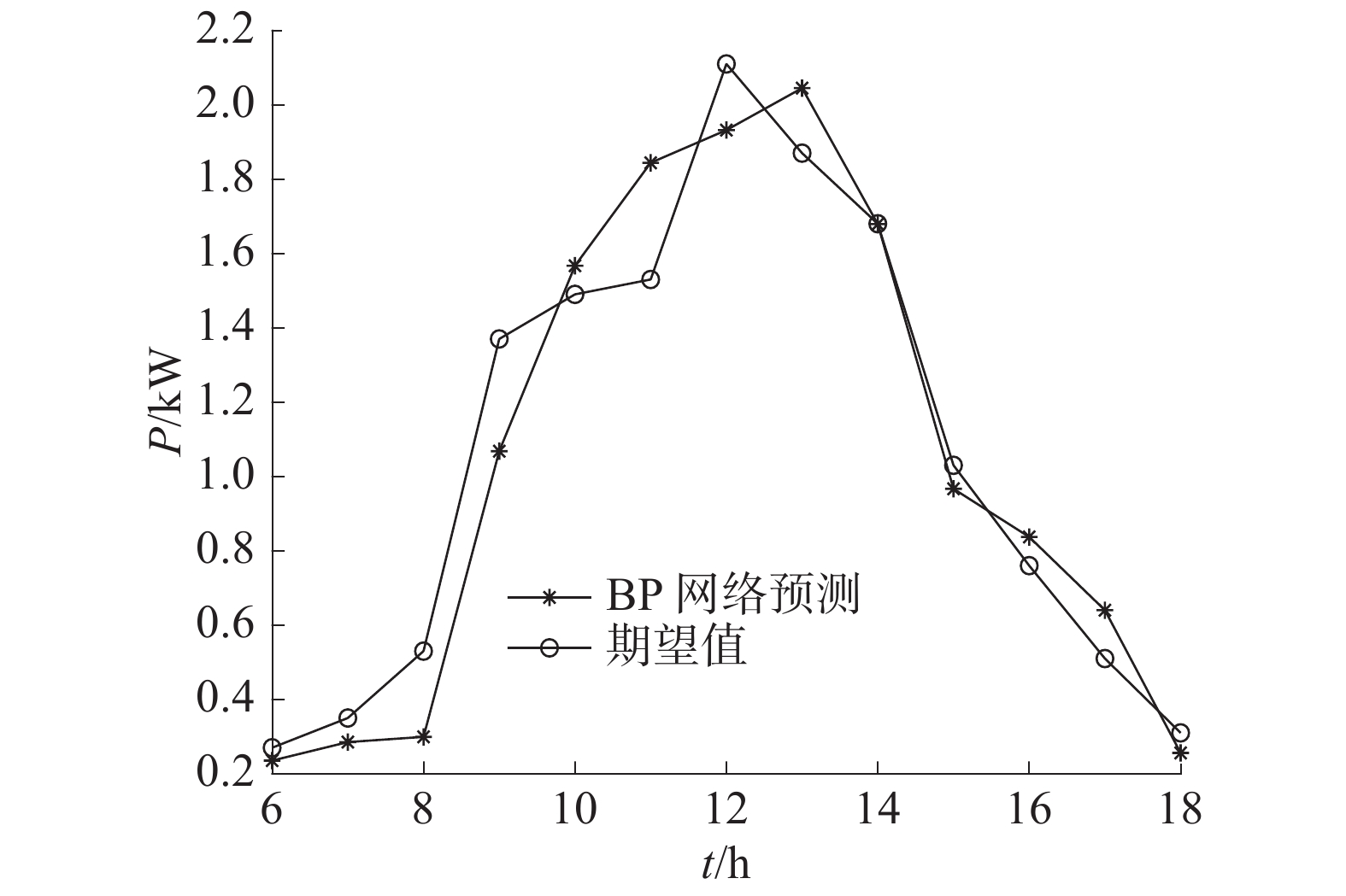Download: 图 2 BP神经网络预测光伏出力数据与期望值对比

3 基于EMD分解的GA-BP光伏出力预测 3.1 遗传算法

3.2 GA-BP模型光伏出力预测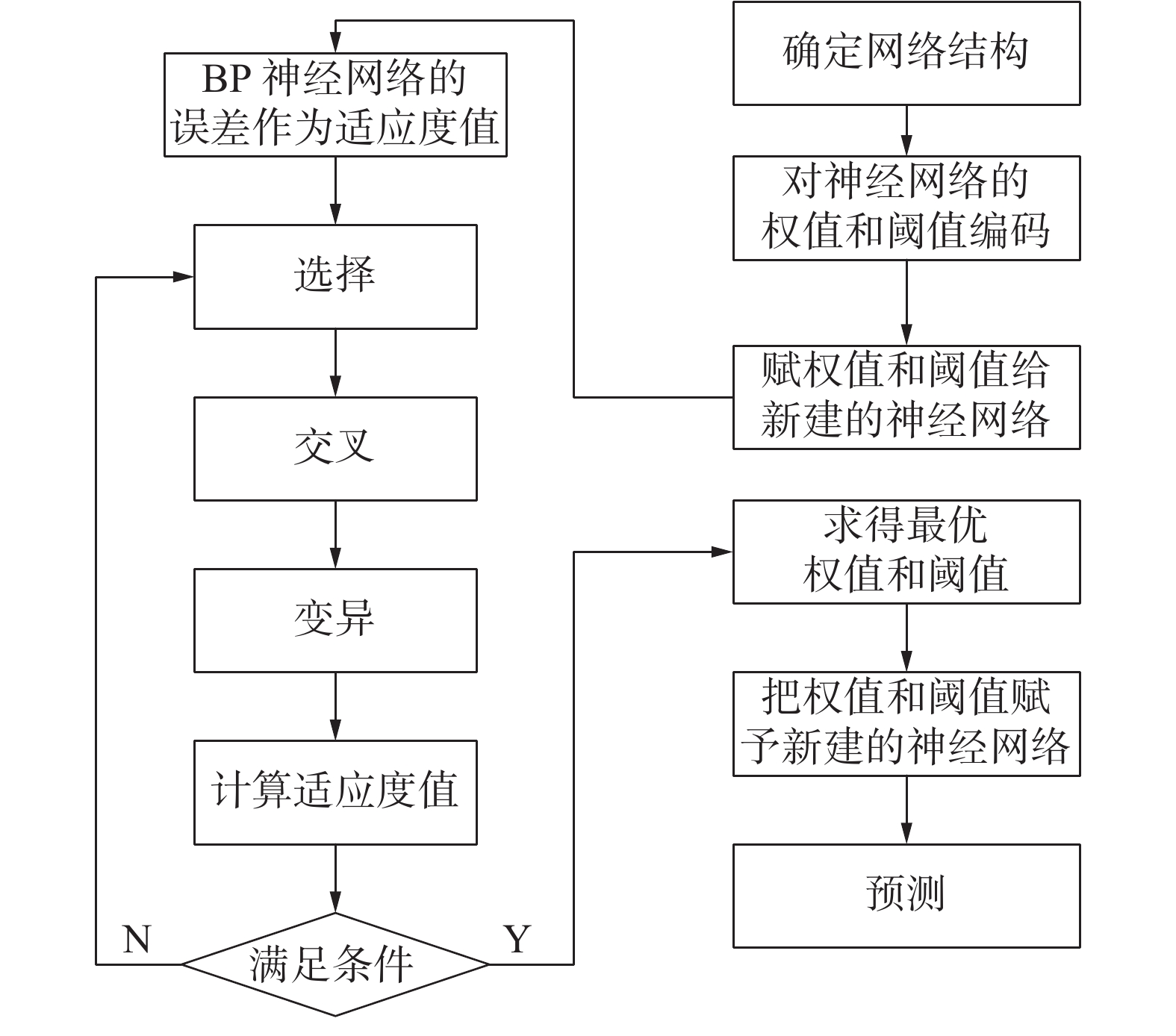Download: 图 3 GA-BP构建流程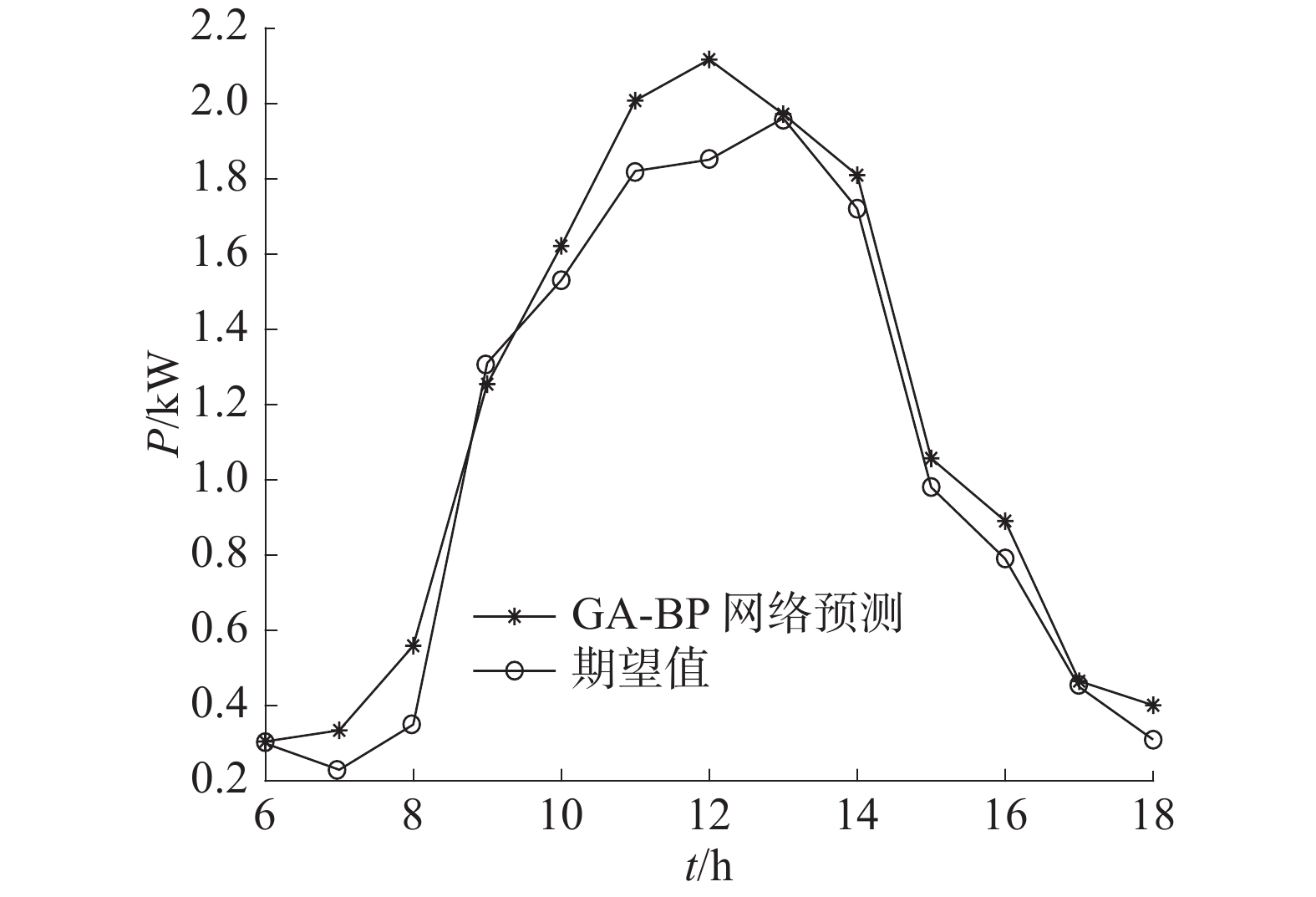Download: 图 4 GA-BP预测光伏出力数据与期望值对比

3.3 基于EMD分解的GA-BP神经网络光伏出力预测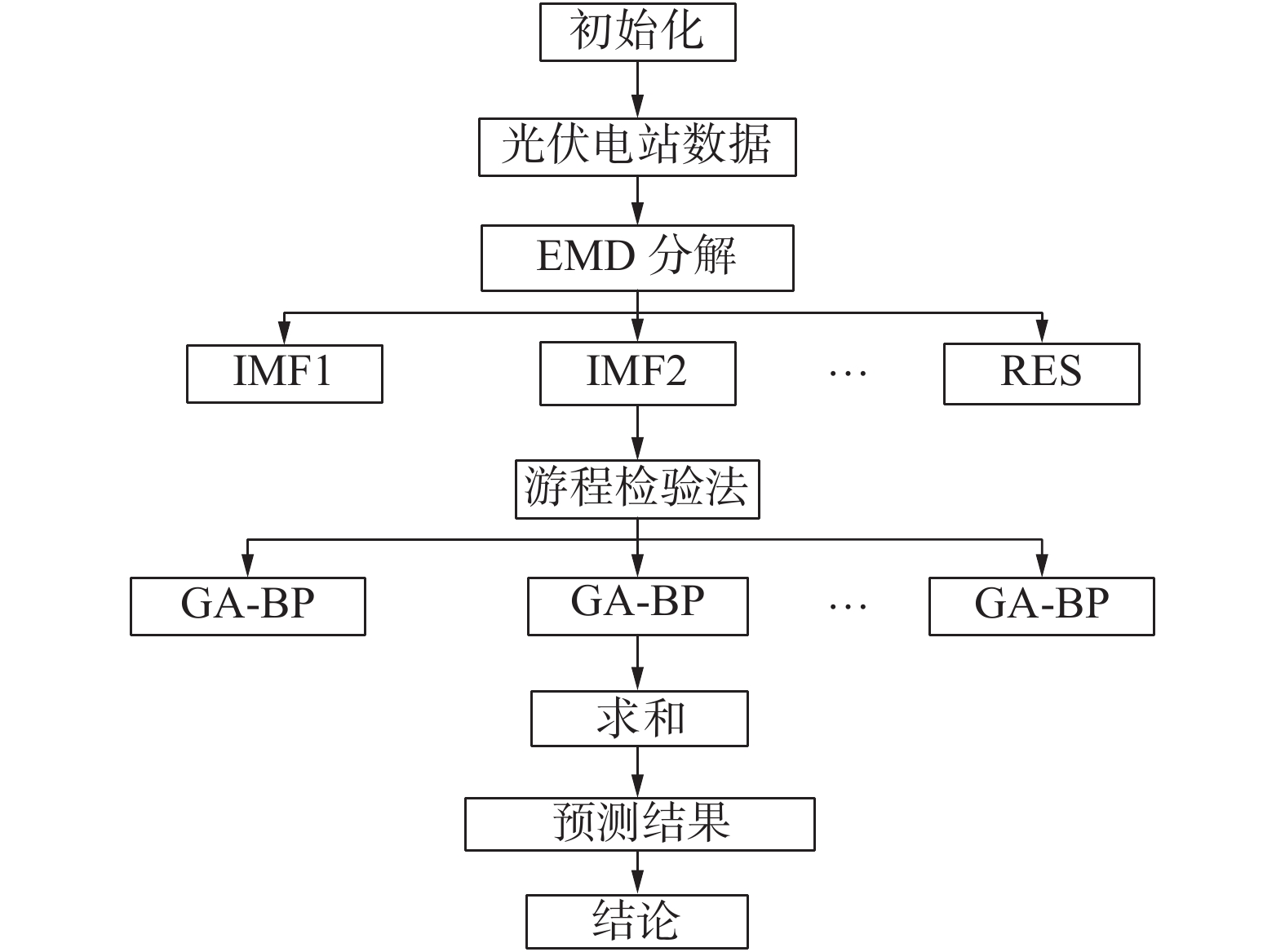Download: 图 5 EMD-GA-BP预测流程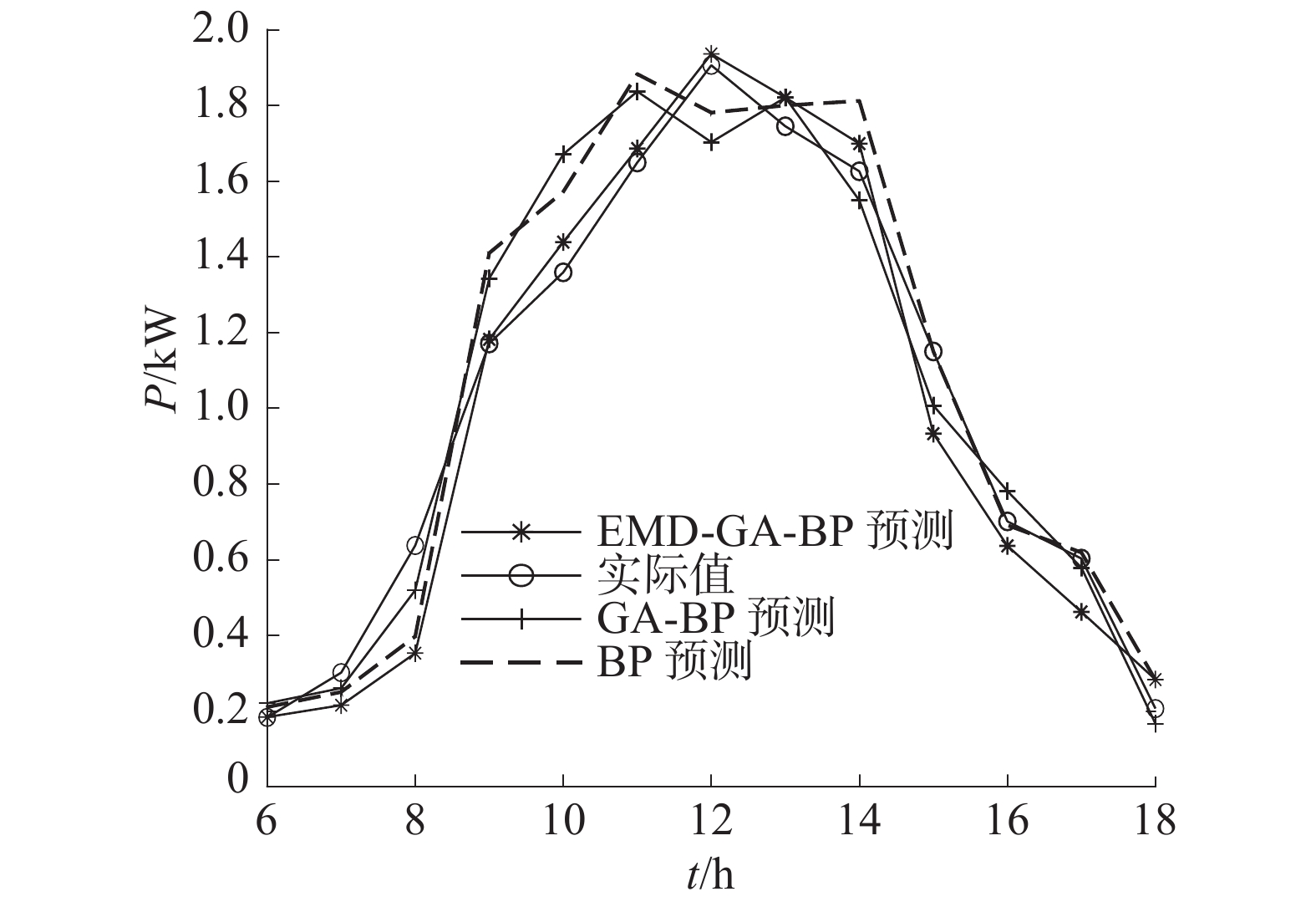Download: 图 6 3种模型预测光伏出力数据与实际值对比表 1 3种模型预测误差对比

4 结论

  应飞祥, 何民, 姜燕波, 等. 光伏发电及其所处电力系统可靠性评估研究进展[J]. 云南民族大学学报(自然科学版), 2019, 28(5): 523-530. (0)  李凯晗. 光伏发电并网逆变器供电稳定性控制研究[J]. 通信电源技术, 2019, 36(2): 26-27. (0)  陈树勇, 鲍海, 吴春洋, 等. 分布式光伏发电并网功率直接控制方法[J]. 中国电机工程学报, 2011, 31(10): 6-11. (0)  EHSAN R M, SIMON S P, VENKATESWARAN P R. Artificial neural network predictor for grid-connected solar photovoltaic installations at atmospheric temperature[C]//Proceedings of 2014 International Conference on Advances in Green Energy. Thiruvananthapuram, India, 2014. (0)  李英姿, 贺琳, 牛进苍. 基于马尔可夫链的光伏并网发电量预测[J]. 太阳能学报, 2014, 35(4): 611-616. DOI:10.3969/j.issn.0254-0096.2014.04.010 (0)  高阳, 张碧玲, 毛京丽, 等. 基于机器学习的自适应光伏超短期出力预测模型[J]. 电网技术, 2015, 39(2): 307-311. (0)  孙祥晟, 陈芳芳, 吴孟礼, 等. 基于EMD分解的AR模型光伏发电预测方法探讨[J]. 电工技术, 2019(11): 8-10. DOI:10.3969/j.issn.1002-1388.2019.11.003 (0)  CHERKASSKY V. The nature of statistical learning theory[J]. IEEE transactions on neural networks, 1997, 8(6): 1564. DOI:10.1109/TNN.1997.641482 (0)  刘晓光, 郝沙沙, 王光磊, 等. 基于自相关特性的经验模态分解微机械陀螺去噪方法[J]. 中国惯性技术学报, 2016, 24(4): 537-541. (0)  杨茂, 张强. 基于集合经验模态分解和相关向量机的风电功率实时预测研究[J]. 太阳能学报, 2016, 37(5): 1093-1099. DOI:10.3969/j.issn.0254-0096.2016.05.002 (0)  王守相, 王亚旻, 刘岩, 等. 基于经验模态分解和ELM神经网络的逐时太阳能辐照量预测[J]. 电力自动化设备, 2014, 34(8): 7-12. DOI:10.3969/j.issn.1006-6047.2014.08.002 (0)  SAHA A K, CHOWDHURY S, CROSSLEY P A. Modeling and performance analysis of a microturbine as a distributed energy resource[J]. IEEE transactions on energy conversion, 2009, 24(2): 529-538. DOI:10.1109/TEC.2009.2016123 (0)  THANGIAH S R, NYGARD K E, JUELL P L. GIDEON: a genetic algorithm system for vehicle routing with time windows[C]//Proceedings the 7th IEEE Conference on Artificial Intelligence Application. Miami Beach, USA, 1991. (0)  郭海湘, 诸克军, 胡杰, 等. GA-BP嵌套算法的理论及应用[J]. 数学的实践与认识, 2008, 38(1): 116-125. (0)